# Selina Solutions Concise Maths Class 10 Chapter 15 Similarity (With Applications to Maps and Models) Exercise 15(C)

The relation between the areas of two similar triangles is the main focus in this exercise. Students having difficulties in understanding any concept can quickly refer to the Selina Solutions for Class 10 Maths, as per their convenience. Experts at BYJU’S have created this valuable tool to help students boost their exam preparations. Further, the solutions to this exercise are available in the Selina Solutions Concise Maths Class 10 Chapter 15 Similarity (With Applications to Maps and Models) Exercise 15(C) PDF in the link below.

## Selina Solutions Concise Maths Class 10 Chapter 15 Similarity (With Applications to Maps and Models) Exercise 15(C) Download PDF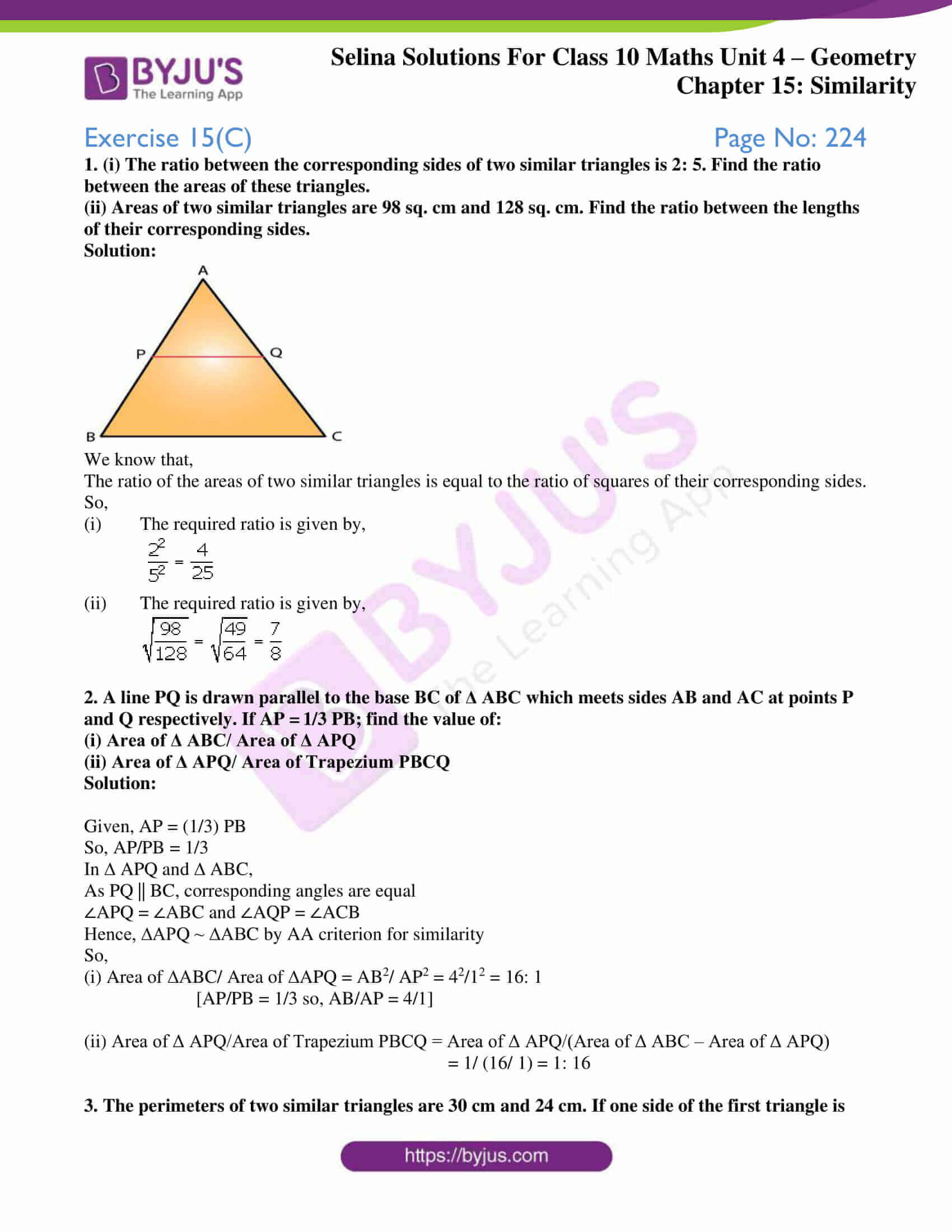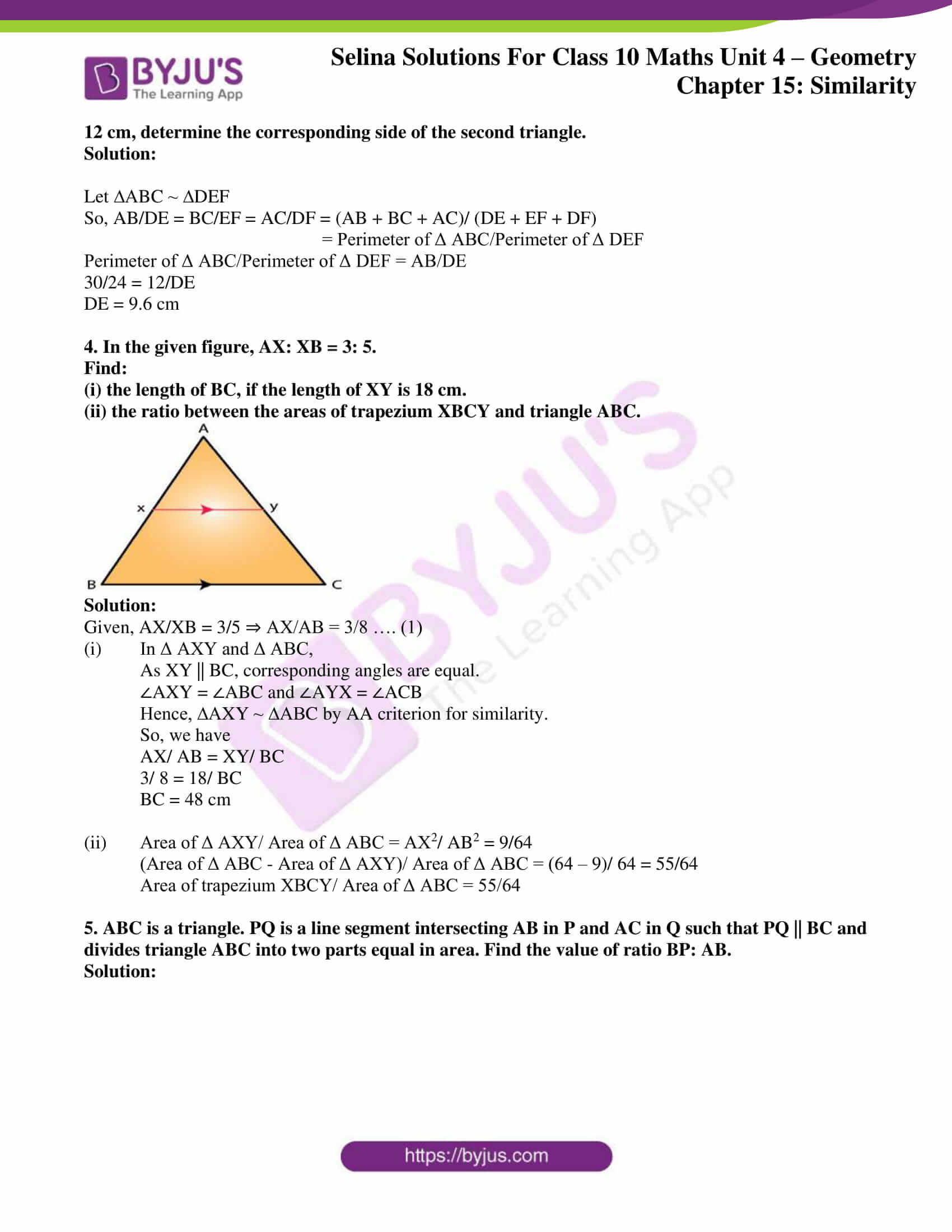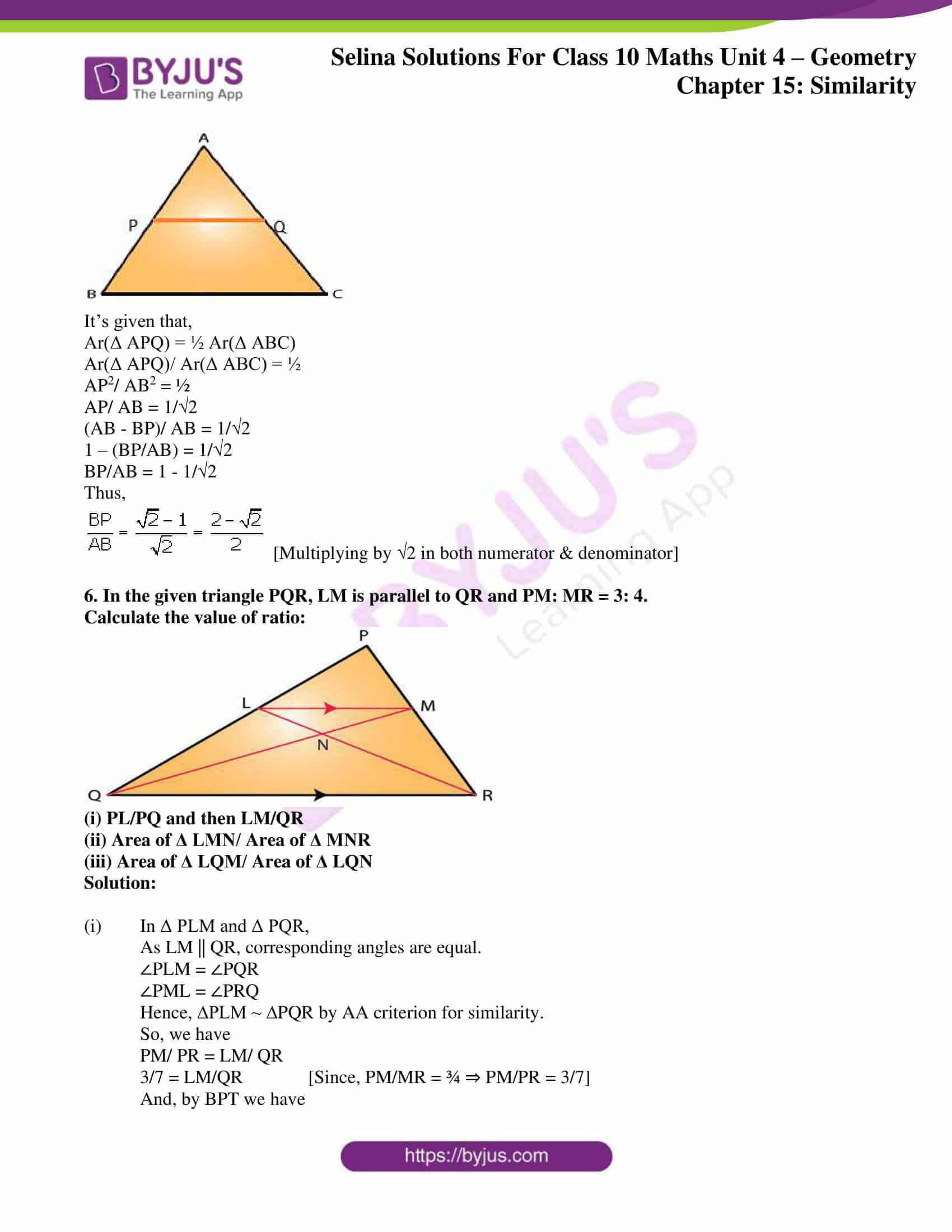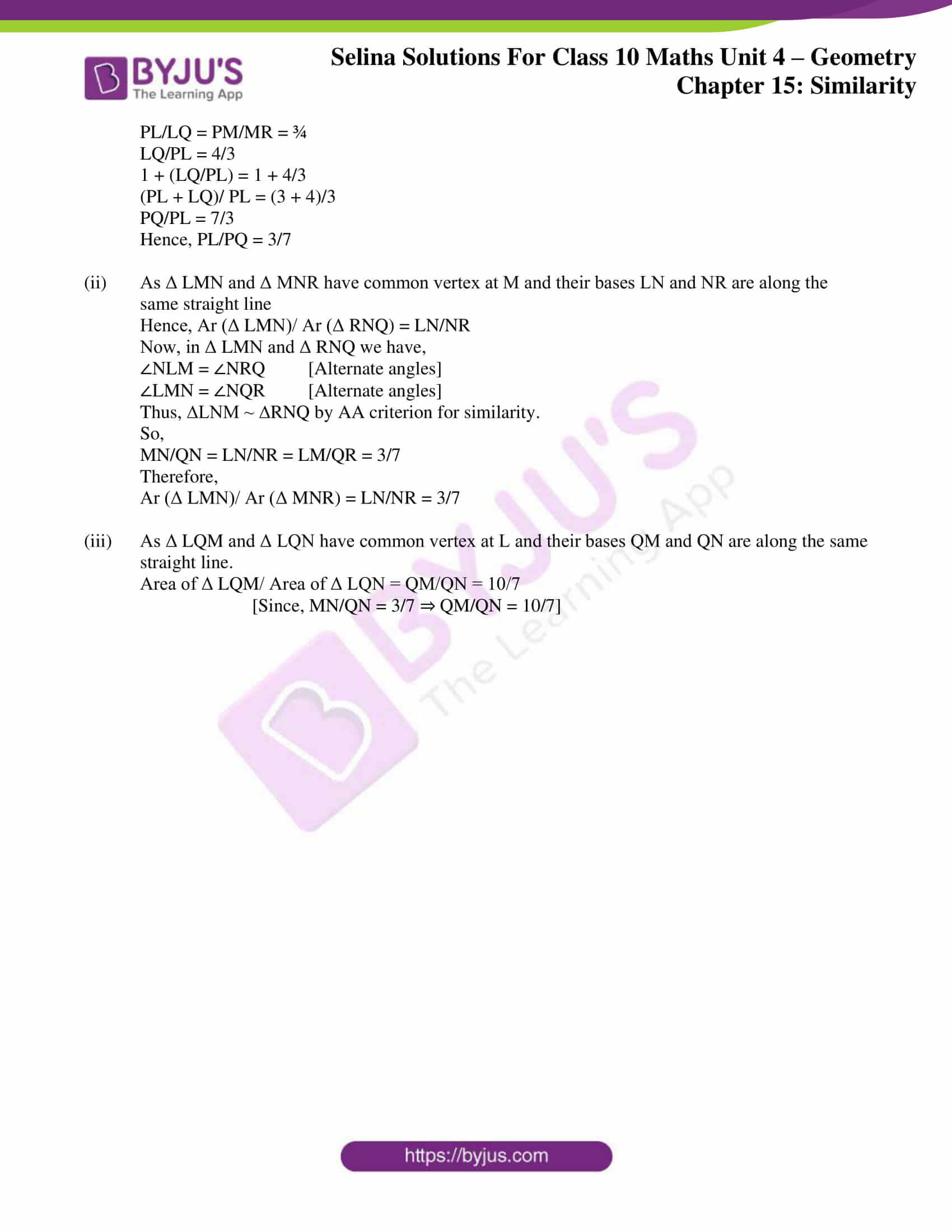## Access Selina Solutions Concise Maths Class 10 Chapter 15 Similarity (With Applications to Maps and Models)

#### Exercise 15(C) Page No: 224

1. (i) The ratio between the corresponding sides of two similar triangles is 2: 5. Find the ratio between the areas of these triangles.

(ii) Areas of two similar triangles are 98 sq. cm and 128 sq. cm. Find the ratio between the lengths of their corresponding sides.

Solution: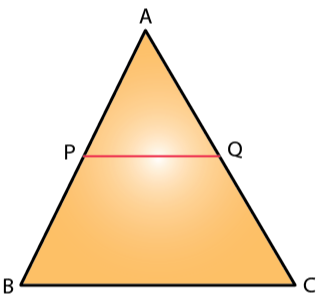We know that,

The ratio of the areas of two similar triangles is equal to the ratio of squares of their corresponding sides.

So,

(i) The required ratio is given by,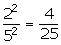(ii) The required ratio is given by,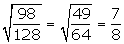2. A line PQ is drawn parallel to the base BC of Δ ABC which meets sides AB and AC at points P and Q respectively. If AP = 1/3 PB; find the value of:

(i) Area of Δ ABC/ Area of Δ APQ

(ii) Area of Δ APQ/ Area of Trapezium PBCQ

Solution:

Given, AP = (1/3) PB

So, AP/PB = 1/3

In ∆ APQ and ∆ ABC,

As PQ || BC, corresponding angles are equal

∠APQ = ∠ABC and ∠AQP = ∠ACB

Hence, ∆APQ ~ ∆ABC by AA criterion for similarity

So,

(i) Area of ∆ABC/ Area of ∆APQ = AB2/ AP2 = 42/12 = 16: 1

[AP/PB = 1/3 so, AB/AP = 4/1]

(ii) Area of Δ APQ/Area of Trapezium PBCQ = Area of Δ APQ/(Area of Δ ABC – Area of Δ APQ)

= 1/ (16/ 1) = 1: 16

3. The perimeters of two similar triangles are 30 cm and 24 cm. If one side of the first triangle is 12 cm, determine the corresponding side of the second triangle.

Solution:

Let ∆ABC ~ ∆DEF

So, AB/DE = BC/EF = AC/DF = (AB + BC + AC)/ (DE + EF + DF)

= Perimeter of Δ ABC/Perimeter of Δ DEF

Perimeter of Δ ABC/Perimeter of Δ DEF = AB/DE

30/24 = 12/DE

DE = 9.6 cm

4. In the given figure, AX: XB = 3: 5.

Find:

(i) the length of BC, if the length of XY is 18 cm.

(ii) the ratio between the areas of trapezium XBCY and triangle ABC.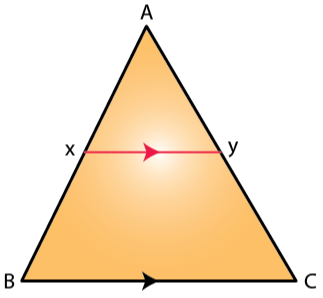Solution:

Given, AX/XB = 3/5 ⇒ AX/AB = 3/8 …. (1)

(i) In Δ AXY and Δ ABC,

As XY || BC, corresponding angles are equal.

∠AXY = ∠ABC and ∠AYX = ∠ACB

Hence, ∆AXY ~ ∆ABC by AA criterion for similarity.

So, we have

AX/ AB = XY/ BC

3/ 8 = 18/ BC

BC = 48 cm

(ii) Area of Δ AXY/ Area of Δ ABC = AX2/ AB2 = 9/64

(Area of Δ ABC – Area of Δ AXY)/ Area of Δ ABC = (64 – 9)/ 64 = 55/64

Area of trapezium XBCY/ Area of Δ ABC = 55/64

5. ABC is a triangle. PQ is a line segment intersecting AB in P and AC in Q such that PQ || BC and divides triangle ABC into two parts equal in area. Find the value of ratio BP: AB.

Solution: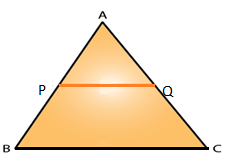It’s given that,

Ar(Δ APQ) = ½ Ar(Δ ABC)

Ar(Δ APQ)/ Ar(Δ ABC) = ½

AP2/ AB2 = ½

AP/ AB = 1/√2

(AB – BP)/ AB = 1/√2

1 – (BP/AB) = 1/√2

BP/AB = 1 – 1/√2

Thus,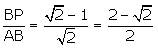[Multiplying by √2 in both numerator & denominator]

6. In the given triangle PQR, LM is parallel to QR and PM: MR = 3: 4.

Calculate the value of ratio: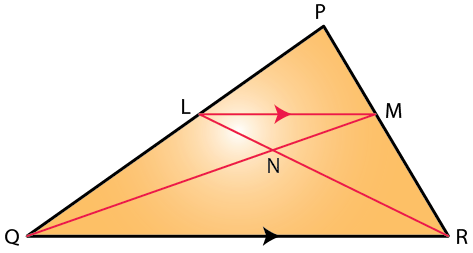(i) PL/PQ and then LM/QR

(ii) Area of Δ LMN/ Area of Δ MNR

(iii) Area of Δ LQM/ Area of Δ LQN

Solution:

(i) In Δ PLM and Δ PQR,

As LM || QR, corresponding angles are equal.

∠PLM = ∠PQR

∠PML = ∠PRQ

Hence, ∆PLM ~ ∆PQR by AA criterion for similarity.

So, we have

PM/ PR = LM/ QR

3/7 = LM/QR [Since, PM/MR = ¾ ⇒ PM/PR = 3/7]

And, by BPT we have

PL/LQ = PM/MR = ¾

LQ/PL = 4/3

1 + (LQ/PL) = 1 + 4/3

(PL + LQ)/ PL = (3 + 4)/3

PQ/PL = 7/3

Hence, PL/PQ = 3/7

(ii) As Δ LMN and Δ MNR have common vertex at M and their bases LN and NR are along the same straight line

Hence, Ar (Δ LMN)/ Ar (Δ RNQ) = LN/NR

Now, in Δ LMN and Δ RNQ we have,

∠NLM = ∠NRQ [Alternate angles]

∠LMN = ∠NQR [Alternate angles]

Thus, ∆LNM ~ ∆RNQ by AA criterion for similarity.

So,

MN/QN = LN/NR = LM/QR = 3/7

Therefore,

Ar (Δ LMN)/ Ar (Δ MNR) = LN/NR = 3/7

(iii) As Δ LQM and Δ LQN have common vertex at L and their bases QM and QN are along the same straight line.

Area of Δ LQM/ Area of Δ LQN = QM/QN = 10/7

[Since, MN/QN = 3/7 ⇒ QM/QN = 10/7]

### Access other exercises of Selina Solutions Concise Maths Class 10 Chapter 15 Similarity (With Applications to Maps and Models)

Exercise 15(A) Solutions

Exercise 15(B) Solutions

Exercise 15(D) Solutions

Exercise 15(E) Solutions